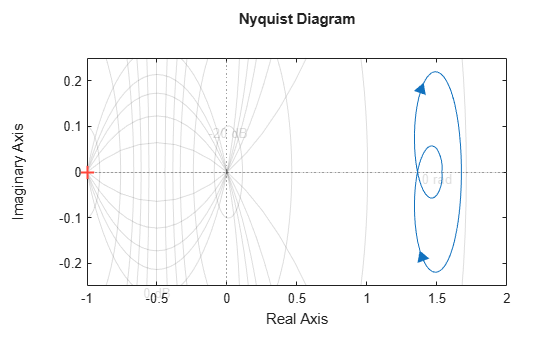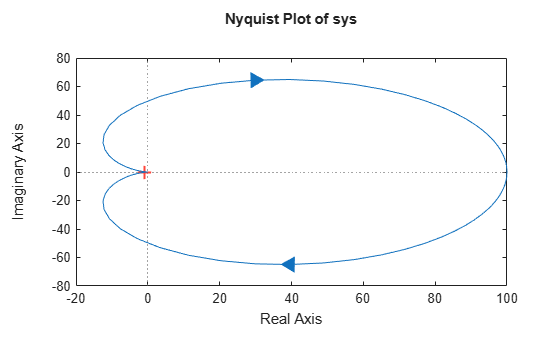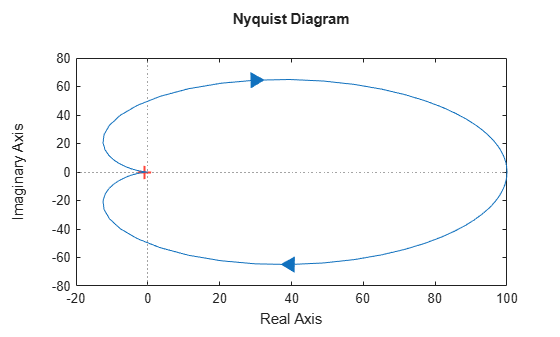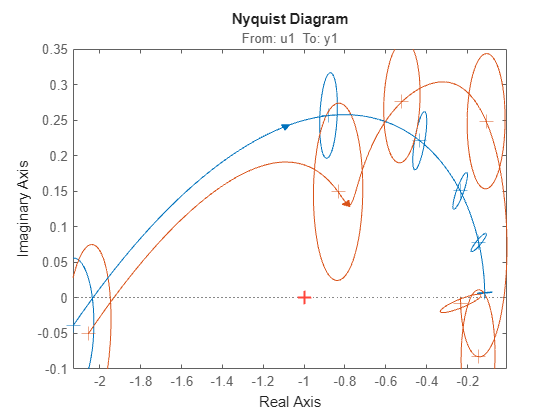# nyquistplot

Nyquist plot with additional plot customization options

## Syntax

``h = nyquistplot(sys)``
``h = nyquistplot(sys1,sys2,...,sysN)``
``h = nyquistplot(sys1,LineSpec1,...,sysN,LineSpecN)``
``h = nyquistplot(___,w)``
``h = nyquistplot(AX,___)``
``h = nyquistplot(___,plotoptions)``

## Description

`nyquistplot` lets you plot the Nyquist diagram of a dynamic system model with a broader range of plot customization options than `nyquist`. You can use `nyquistplot` to obtain the plot handle and use it to customize the plot, such as modify the axes labels, limits and units. You can also use `nyquistplot` to draw a Nyquist diagram on an existing set of axes represented by an axes handle. To customize an existing Nyquist plot using the plot handle:

1. Obtain the plot handle

2. Use `getoptions` to obtain the option set

3. Update the plot using `setoptions` to modify the required options

For more information, see Customizing Response Plots from the Command Line. To create Nyquist plots with default options or to extract the standard deviation, real and imaginary parts of the frequency response data, use `nyquist`.

example

````h = nyquistplot(sys)` plots the Nyquist plot of the dynamic system model `sys` and returns the plot handle `h` to the plot. You can use this handle `h` to customize the plot with the `getoptions` and `setoptions` commands. If `sys` is a multi-input, multi-output (MIMO) model, then `nyquistplot` produces a grid of Nyquist plots, each plot displaying the frequency response of one I/O pair.```

example

````h = nyquistplot(sys1,sys2,...,sysN)` plots the Nyquist plot of multiple dynamic systems `sys1,sys2,…,sysN` on the same plot. All systems must have the same number of inputs and outputs to use this syntax.```

example

````h = nyquistplot(sys1,LineSpec1,...,sysN,LineSpecN)` sets the line style, marker type, and color for the Nyquist plot of each system. All systems must have the same number of inputs and outputs to use this syntax.```

example

````h = nyquistplot(___,w)` plots Nyquist diagram for frequencies specified by the frequencies in `w`.If `w` is a cell array of the form `{wmin,wmax}`, then `nyquistplot` plots the Nyquist diagram at frequencies ranging between `wmin` and `wmax`.If `w` is a vector of frequencies, then `nyquistplot` plots the Nyquist diagram at each specified frequency.You can use `w` with any of the input-argument combinations in previous syntaxes.See `logspace` to generate logarithmically spaced frequency vectors.```
````h = nyquistplot(AX,___)` plots the Nyquist plot on the `Axes` object in the current figure with the handle `AX`.```

example

````h = nyquistplot(___,plotoptions)` plots the Nyquist plot with the options set specified in `plotoptions`. You can use these options to customize the Nyquist plot appearance using the command line. Settings you specify in `plotoptions` overrides the preference settings in the MATLAB® session in which you run `nyquistplot`. Therefore, this syntax is useful when you want to write a script to generate multiple plots that look the same regardless of the local preferences.```

## Examples

collapse all

For this example, use the plot handle to change the phase units to radians and to turn the grid on.

Generate a random state-space model with 5 states and create the Nyquist diagram with plot handle `h`.

```rng("default") sys = rss(5); h = nyquistplot(sys);```Change the phase units to radians and turn on the grid. To do so, edit properties of the plot handle, `h` using `setoptions`.

`setoptions(h,'PhaseUnits','rad','Grid','on');`The Nyquist plot automatically updates when you call `setoptions`.

Alternatively, you can also use the `nyquistoptions` command to specify the required plot options. First, create an options set based on the toolbox preferences.

`plotoptions = nyquistoptions('cstprefs');`

Change properties of the options set by setting the phase units to radians and enabling the grid.

```plotoptions.PhaseUnits = 'rad'; plotoptions.Grid = 'on'; nyquistplot(sys,plotoptions);```You can use the same option set to create multiple Nyquist plots with the same customization. Depending on your own toolbox preferences, the plot you obtain might look different from this plot. Only the properties that you set explicitly, in this example `PhaseUnits` and `Grid`, override the toolbox preferences.

Create a Nyquist plot of a dynamic system model and store a handle to the plot.

```sys = tf(100,[1,2,1]); h = nyquistplot(sys);```Change the plot title to read "Nyquist Plot of sys." To do so, use `getoptions` to extract the existing plot options from the plot handle `h`.

`opt = getoptions(h)`
```opt = FreqUnits: 'rad/s' MagUnits: 'dB' PhaseUnits: 'deg' ShowFullContour: 'on' ConfidenceRegionNumberSD: 1 ConfidenceRegionDisplaySpacing: 5 IOGrouping: 'none' InputLabels: [1x1 struct] OutputLabels: [1x1 struct] InputVisible: {'on'} OutputVisible: {'on'} Title: [1x1 struct] XLabel: [1x1 struct] YLabel: [1x1 struct] TickLabel: [1x1 struct] Grid: 'off' GridColor: [0.1500 0.1500 0.1500] XLim: {[-20 100]} YLim: {[-80 80]} XLimMode: {'auto'} YLimMode: {'auto'} ```

The `Title` option is a structure with several fields.

`opt.Title`
```ans = struct with fields: String: 'Nyquist Diagram' FontSize: 11 FontWeight: 'bold' FontAngle: 'normal' Color: [0 0 0] Interpreter: 'tex' ```

Change the `String` field of the `Title` structure, and use `setoptions` to apply the change to the plot.

```opt.Title.String = 'Nyquist Plot of sys'; setoptions(h,opt)```Plot the Nyquist frequency response of a dynamic system. Assign a variable name to the plot handle so that you can access it for further manipulation.

```sys = tf(100,[1,2,1]); h = nyquistplot(sys);```Zoom in on the critical point, (–1,0). You can do so interactively by right-clicking on the plot and selecting Zoom on (-1,0). Alternatively, use the `zoomcp` command on the plot handle `h`.

`zoomcp(h)`Compare the frequency responses of identified state-space models of order 2 and 6 along with their `1-std` confidence regions rendered at every 50th frequency sample.

Load the identified model data and estimate the state-space models using `n4sid`. Then, plot the Nyquist diagram.

```load iddata1 sys1 = n4sid(z1,2); sys2 = n4sid(z1,6); w = linspace(10,10*pi,256); h = nyquistplot(sys1,sys2,w);```Both models produce about 76% fit to data. However, `sys2` shows higher uncertainty in its frequency response, especially close to Nyquist frequency as shown by the plot. To see this, show the confidence region at a subset of the points at which the Nyquist response is displayed.

```setoptions(h,'ConfidenceRegionDisplaySpacing',50,... 'ShowFullContour','off');```To turn on the confidence region display, right-click the plot and select Characteristics > Confidence Region.For this example, consider a MIMO state-space model with 3 inputs, 3 outputs and 3 states. Create a Nyquist plot, display only the partial contour and turn the grid on.

Create the MIMO state-space model `sys_mimo`.

```J = [8 -3 -3; -3 8 -3; -3 -3 8]; F = 0.2*eye(3); A = -J\F; B = inv(J); C = eye(3); D = 0; sys_mimo = ss(A,B,C,D); size(sys_mimo)```
```State-space model with 3 outputs, 3 inputs, and 3 states. ```

Create a Nyquist plot with plot handle `h` and use `getoptions` for a list of the options available.

`h = nyquistplot(sys_mimo);``p = getoptions(h)`
```p = FreqUnits: 'rad/s' MagUnits: 'dB' PhaseUnits: 'deg' ShowFullContour: 'on' ConfidenceRegionNumberSD: 1 ConfidenceRegionDisplaySpacing: 5 IOGrouping: 'none' InputLabels: [1x1 struct] OutputLabels: [1x1 struct] InputVisible: {3x1 cell} OutputVisible: {3x1 cell} Title: [1x1 struct] XLabel: [1x1 struct] YLabel: [1x1 struct] TickLabel: [1x1 struct] Grid: 'off' GridColor: [0.1500 0.1500 0.1500] XLim: {3x1 cell} YLim: {3x1 cell} XLimMode: {3x1 cell} YLimMode: {3x1 cell} ```

Use `setoptions` to update the plot with the requires customization.

`setoptions(h,'ShowFullContour','off','Grid','on');`The Nyquist plot automatically updates when you call `setoptions`. For MIMO models, `nyquistplot` produces an array of Nyquist diagrams, each plot displaying the frequency response of one I/O pair.

## Input Arguments

collapse all

Dynamic system, specified as a SISO or MIMO dynamic system model or array of dynamic system models. Dynamic systems that you can use include:

• Continuous-time or discrete-time numeric LTI models, such as `tf`, `zpk`, or `ss` models.

• Sparse state-space models, such as `sparss` or `mechss` models. Frequency grid `w` must be specified for sparse models.

• Generalized or uncertain LTI models such as `genss` or `uss` (Robust Control Toolbox) models. (Using uncertain models requires Robust Control Toolbox™ software.)

• For tunable control design blocks, the function evaluates the model at its current value to plot the frequency response data.

• For uncertain control design blocks, the function plots the nominal value and random samples of the model.

• Frequency-response data models such as `frd` models. For such models, the function plots the Nyquist plot at frequencies defined in the model.

• Identified LTI models, such as `idtf` (System Identification Toolbox), `idss` (System Identification Toolbox), or `idproc` (System Identification Toolbox) models. (Using identified models requires System Identification Toolbox™ software.)

If `sys` is an array of models, the function plots the Nyquist responses of all models in the array on the same axes.

Line style, marker, and color, specified as a character vector or string containing symbols. The symbols can appear in any order. You do not need to specify all three characteristics (line style, marker, and color). For example, if you omit the line style and specify the marker, then the plot shows only the marker and no line.

Example: `'--or'` is a red dashed line with circle markers

Line StyleDescription
`-`Solid line
`--`Dashed line
`:`Dotted line
`-.`Dash-dot line
MarkerDescription
`'o'`Circle
`'+'`Plus sign
`'*'`Asterisk
`'.'`Point
`'x'`Cross
`'_'`Horizontal line
`'|'`Vertical line
`'s'`Square
`'d'`Diamond
`'^'`Upward-pointing triangle
`'v'`Downward-pointing triangle
`'>'`Right-pointing triangle
`'<'`Left-pointing triangle
`'p'`Pentagram
`'h'`Hexagram
ColorDescription

`y`

yellow

`m`

magenta

`c`

cyan

`r`

red

`g`

green

`b`

blue

`w`

white

`k`

black

Target axes, specified as an `Axes` or `UIAxes` object. If you do not specify the axes and if the current axes are Cartesian axes, then `nyquistplot` plots on the current axes.

Nyquist plot options set, specified as a `NyquistPlotOptions` object. You can use this option set to customize the Nyquist plot appearance. Use `nyquistoptions` to create the option set. Settings you specify in `plotoptions` overrides the preference settings in the MATLAB session in which you run `nyquistplot`. Therefore, `plotoptions` is useful when you want to write a script to generate multiple plots that look the same regardless of the local preferences.

For the list of available options, see `nyquistoptions`.

Frequencies at which to compute and plot Nyquist response, specified as the cell array `{wmin,wmax}` or as a vector of frequency values.

• If `w` is a cell array of the form `{wmin,wmax}`, then the function computes the response at frequencies ranging between `wmin` and `wmax`.

• If `w` is a vector of frequencies, then the function computes the response at each specified frequency. For example, use `logspace` to generate a row vector with logarithmically spaced frequency values.

Specify frequencies in units of `rad/TimeUnit`, where `TimeUnit` is the `TimeUnit` property of the model.

## Output Arguments

collapse all

Plot handle, returned as a `handle` object. Use the handle `h` to get and set the properties of the Nyquist plot using `getoptions` and `setoptions`. For the list of available options, see the Properties and Values Reference section in Customizing Response Plots from the Command Line.

## Tips

• There are two zoom options available from the right-click menu that apply specifically to Nyquist plots:

• Full View — Clips unbounded branches of the Nyquist plot, but still includes the critical point (–1, 0).

• Zoom on (-1,0) — Zooms around the critical point (–1,0). To access critical-point zoom programmatically, use the `zoomcp` command. See Zoom on Critical Point.

• To activate data markers that display the real and imaginary values at a given frequency, click anywhere on the curve. The following figure shows a Nyquist plot with a data marker.